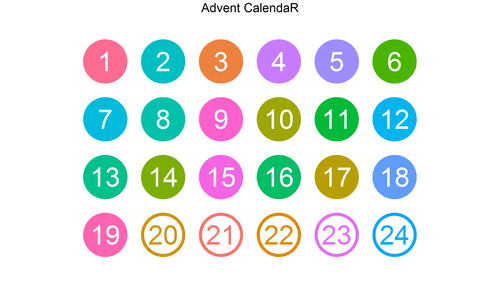[This article was first published on is.R(), and kindly contributed to R-bloggers]. (You can report issue about the content on this page here)
Want to share your content on R-bloggers? click here if you have a blog, or here if you don't.Given a random list of words, can you find which has the lowest or highest numerical value when we apply a basic number:letter cipher?

A while back I asked David how he would solve this problem:

http://projecteuler.net/problem=42

Today’s post shows how to take a vector of words, parse them into each of the individual letters comprising the word, and calculate the sum of the value of the word using A=1, B=2, …, Z=26.

Enjoy!

P.S. The code can be modified to find the answer (162) to:

http://projecteuler.net/problem=42